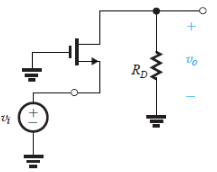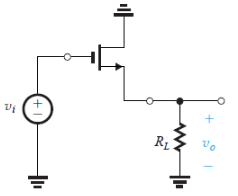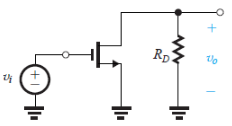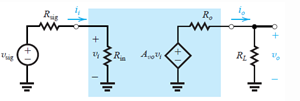Courses

# Test: Basic MOSFET Amplifier Configurations

## 10 Questions MCQ Test Electronic Devices | Test: Basic MOSFET Amplifier Configurations

Description
This mock test of Test: Basic MOSFET Amplifier Configurations for Electrical Engineering (EE) helps you for every Electrical Engineering (EE) entrance exam. This contains 10 Multiple Choice Questions for Electrical Engineering (EE) Test: Basic MOSFET Amplifier Configurations (mcq) to study with solutions a complete question bank. The solved questions answers in this Test: Basic MOSFET Amplifier Configurations quiz give you a good mix of easy questions and tough questions. Electrical Engineering (EE) students definitely take this Test: Basic MOSFET Amplifier Configurations exercise for a better result in the exam. You can find other Test: Basic MOSFET Amplifier Configurations extra questions, long questions & short questions for Electrical Engineering (EE) on EduRev as well by searching above.
QUESTION: 1

### In which of the following configuration does a MOSFET works as an amplifier?

Solution:

There are three basic configurations for connecting the MOSFET as an amplifier. Each of these configurations is obtained by connecting one of the three MOSFET terminals to ground, thus creating a two-port network with the grounded terminal being common to the input and output ports.

QUESTION: 2

### The MOSFET in the following circuit is in which configuration?​Solution:

It is the circuit for Common gate configuration.

QUESTION: 3

### The MOSFET in the following circuit is in which configuration?Solution:

It is the circuit for Common drain configuration.

QUESTION: 4

The MOSFET in the following circuit is in which configuration?[/expand]​Solution:

It is the circuit for Common source configuration.

QUESTION: 5

(Q.5-Q.10) Reference circuit for Q.5-Q.10 The circuit below is the characterization for the amplifier as a functional block.If the value of Rin for the common source configuration is R1 and that for common source with a source resistance configuration is R2 ideally. The ratio of R1/R2 will be

Solution:

Ideally both must have infinite resistance.

QUESTION: 6

Which is true for the value of Avo for common source (Represented by A1) and common source with a source resistance (represented by A2).

Solution:

A1 = -gmRD and A2 = -gmRD/1+gmRS
Reference circuit for Common source configuration

QUESTION: 7

Which of the following is true for the voltage gain (AV) for the common source configuration (represented by A1) and the common gate configuration (represented by A2)?

Solution:
QUESTION: 8

The value of the voltage gain (Av) for the common source with source resistance (represented by A1) and common gate configuration (represented by A2) are related to each other by

Solution:
QUESTION: 9

In which of the following configuration is the input resistance (Ri) not equal to zero ideally?

Solution:
QUESTION: 10

Which of the following has AVO independent of the circuit elements?

Solution:

AVO = 1 source follower.